# Problem 6: Let B = {V1, V2, ..., Un} be a set of vectors in R",...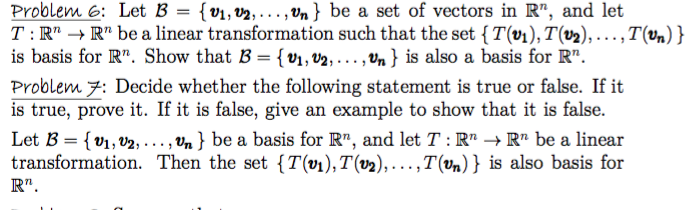Problem 6: Let B = {V1, V2, ..., Un} be a set of vectors in R", and let T:R" → R" be a linear transformation such that the set {T(01), T(V2), ...,T(Un) } is basis for R". Show that B = {01, V2, ..., Un } is also a basis for R". Problem 7: Decide whether the following statement is true or false. If it is true, prove it. If it is false, give an example to show that it is false. Let B = {V1, V2, ..., Vn} be a basis for R", and let T : RM + Rr be a linear transformation. Then the set {T(01), T(v2),...,T(Un) } is also basis for R".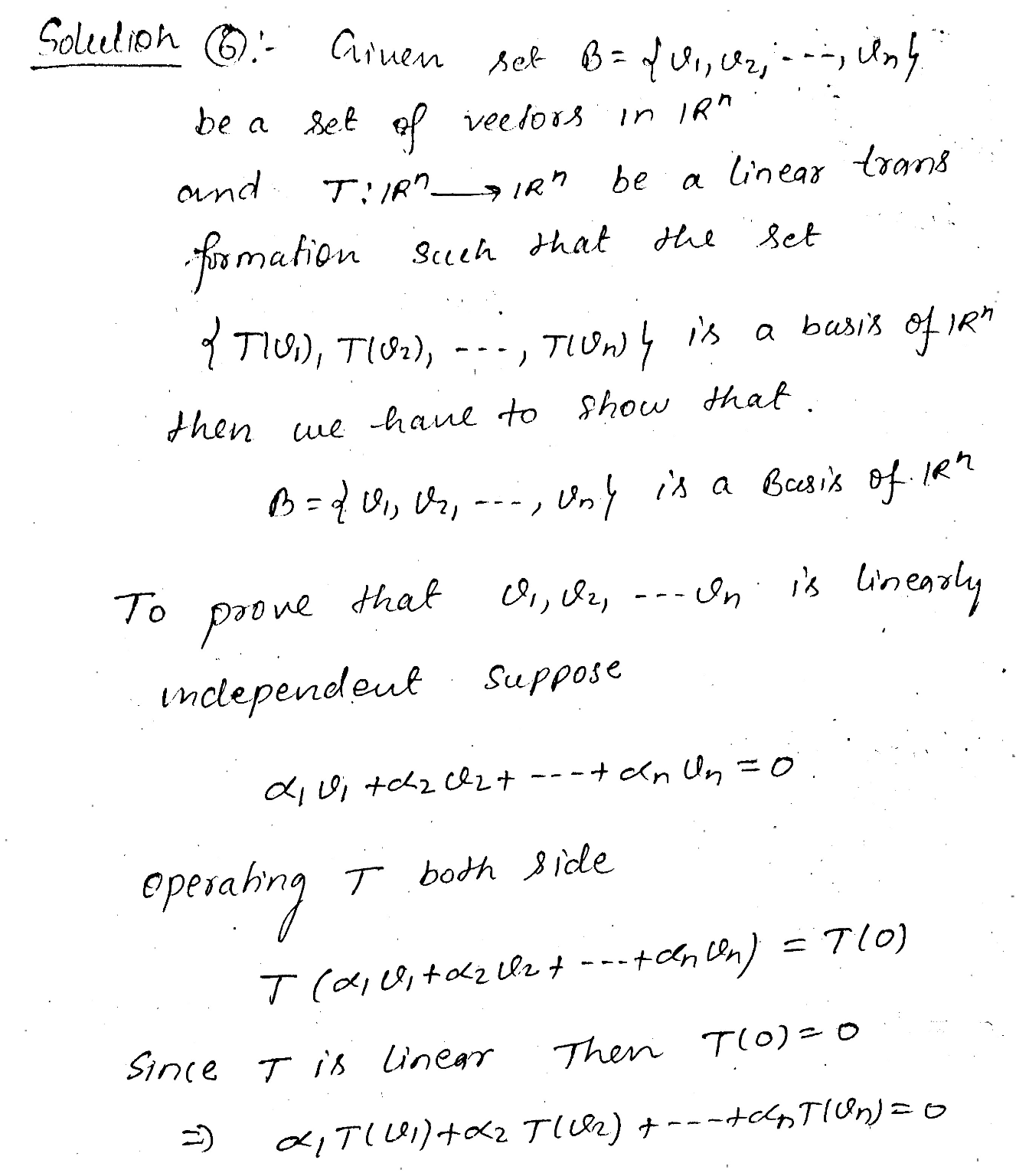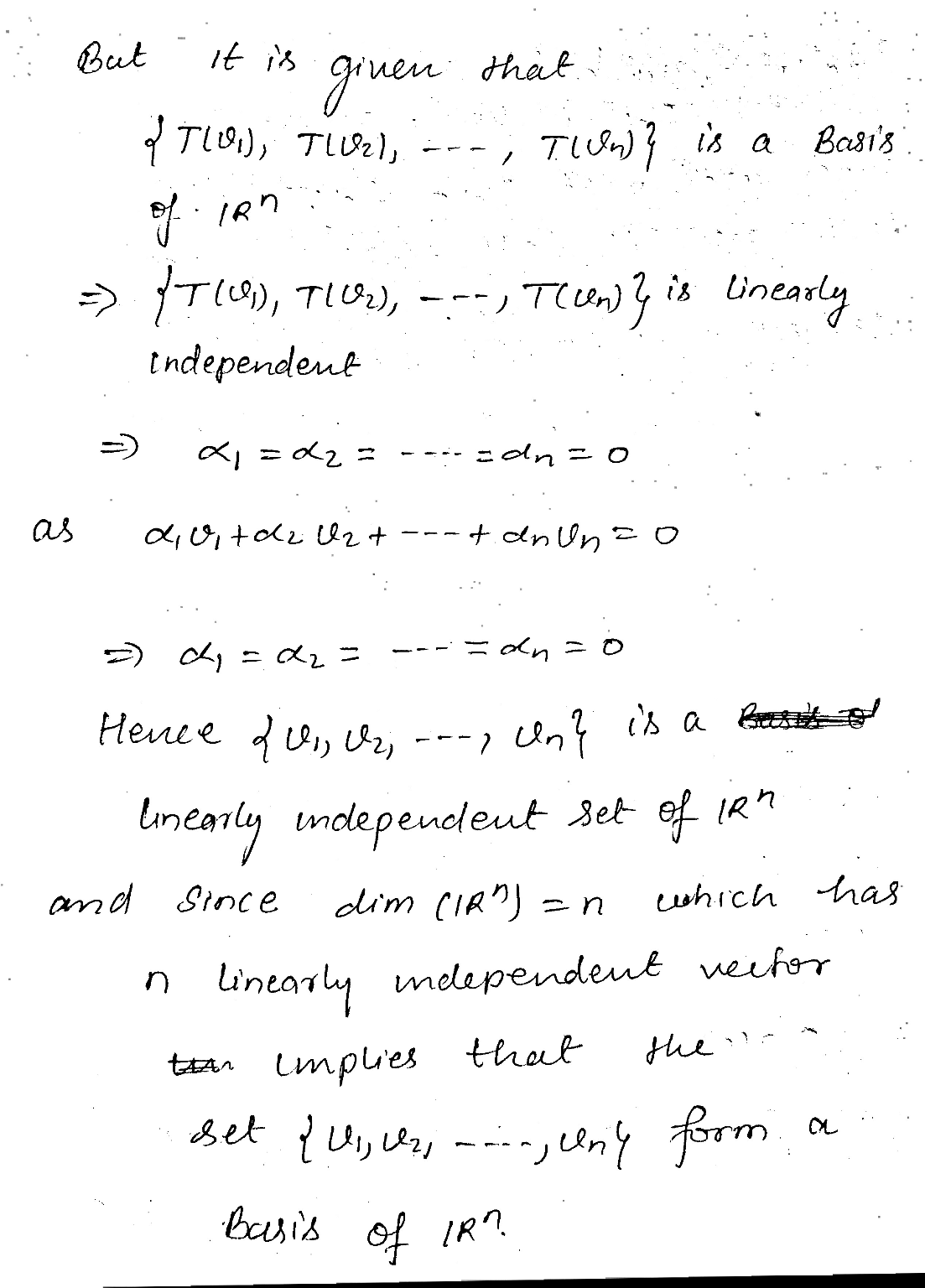##### Add Answer of: Problem 6: Let B = {V1, V2, ..., Un} be a set of vectors in R",...
Similar Homework Help Questions
• ### Problem 5: Let V and W be vector spaces and let B = {V1, V2, ...,...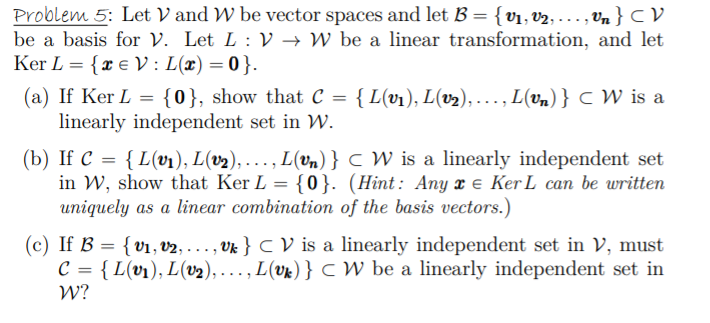Problem 5: Let V and W be vector spaces and let B = {V1, V2, ..., Un} CV be a basis for V. Let L :V + W be a linear transformation, and let Ker L = {2 € V: L(x)=0}. (a) If Ker L = {0}, show that C = {L(v1), L(02), ..., L(vn) } CW is a linearly independent set in W. (b) If C = {L(01), L(V2),..., L(Un)} C W is a linearly independent set in W,...

• ### 7. Let T : V → W be a linear transformation, and let v1,v2,...,vn be vectors...

7. Let T : V → W be a linear transformation, and let v1,v2,...,vn be vectors in V. Suppose that T (v1), T (v2), . . . , T (vn) are linearly independent. Show that v1, v2, . . . , vn are linearly independent.

• ### 6. Given the points A = (0,0), B = (5,1), C = (2,6) on the plane....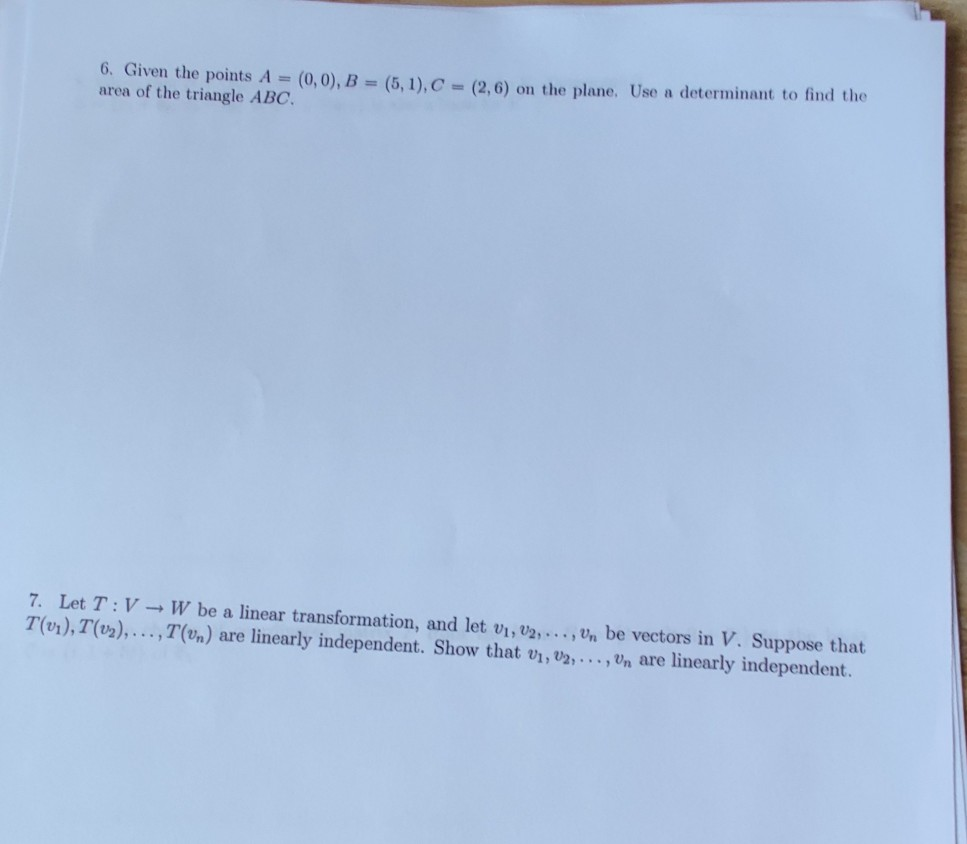6. Given the points A = (0,0), B = (5,1), C = (2,6) on the plane. Use a determinant to find the area of the triangle ABC. 7. Let T:V - W be a linear transformation, and let V1, V2, ..., Un be vectors in V. Suppose that T(vi), T(02),...,T(un) are linearly independent. Show that V1, V2, ..., Vn are linearly independent. 3. Given that 8 - ...) is a basis for a vector space V. Determine if 3 -...

• ### 6. Given the points A = (0,0), B = (5,1), C = (2,6) on the plane....6. Given the points A = (0,0), B = (5,1), C = (2,6) on the plane. Use a determinant to find the area of the triangle ABC. 7. Let T:V - W be a linear transformation, and let V1, V2, ..., Un be vectors in V. Suppose that T(vi), T(02),...,T(un) are linearly independent. Show that V1, V2, ..., Vn are linearly independent.

• ### 6. Given the points A = (0,0), B = (5,1), C = (2,6) on the plane....6. Given the points A = (0,0), B = (5,1), C = (2,6) on the plane. Use a determinant to find the area of the triangle ABC. 7. Let T:V - W be a linear transformation, and let V1, V2, ..., Un be vectors in V. Suppose that T(vi), T(02),...,T(un) are linearly independent. Show that V1, V2, ..., Vn are linearly independent.

• ### (1) Let S (v1, V2,..., Vn be a set of vectors in a vector space V....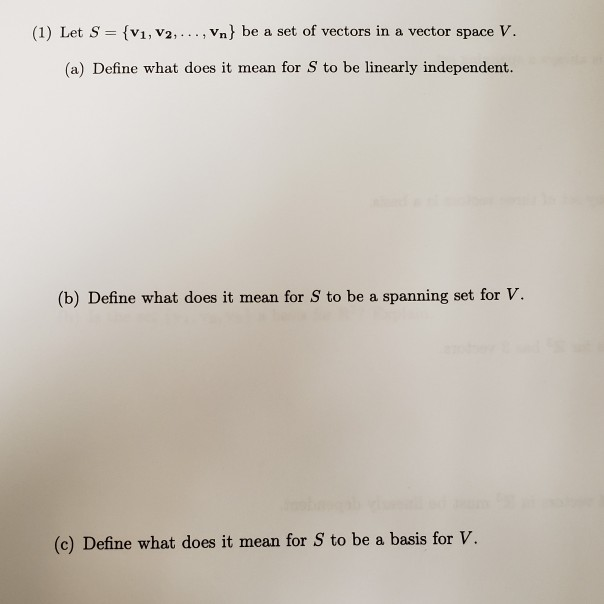(1) Let S (v1, V2,..., Vn be a set of vectors in a vector space V. (a) Define what does it mean for S to be linearly independent. (b) Define what does it mean for S to be a spanning set for V. (c) Define what does it mean for S to be a basis for v.

• ### Linear Algebra problem(1)

Recall that linear transformation T: R^n -> R^n is called an esometry if <T(x), T(y)>=<x,y> for all vectors x and y in R^n. Thus an isometry is alinear transformation that preserves inner products.(a) Show that if B={v1,v2,.....,vn} form an orthonormal basis for R^n, then T(v1),T(v2),....T(vn) form an orthonormal basis for R^n, and conclude that the matrixfor T with respect to B is orthogonal.(b) Show that if B={v1,v2,.....,vn} form an orthonormal basis for V and the matrix [T]B of a linear...

• ### 7. Let T:V : - W be a linear transformation, and let vi, U2,..., Un be...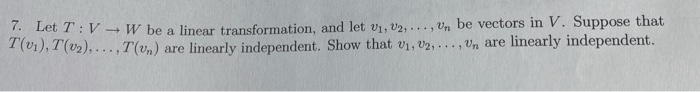7. Let T:V : - W be a linear transformation, and let vi, U2,..., Un be vectors in V. Suppose that T(01), T (v2),..., 1 (un) are linearly independent. Show that 01, V2, ..., Un are linearly independent.

• ### Prove Lemma a) Fix a basis {v1, v2, . . . , vn} for an n-dimensional...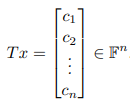Prove Lemma a) Fix a basis {v1, v2, . . . , vn} for an n-dimensional vector space V. Define a linear operator T : V → Fn in the following way: For each x = Σni=1 civi ∈ V, define . Then T is a linear operator. b) Let T be a linear operator from V to W. Suppose that {v1, v2, . . . , vn} is a basis for V and {w1, w2, . . . ,...

• ### Problem 4. Let GL2(R) be the vector space of 2 x 2 square matrices with usual matrix addition and...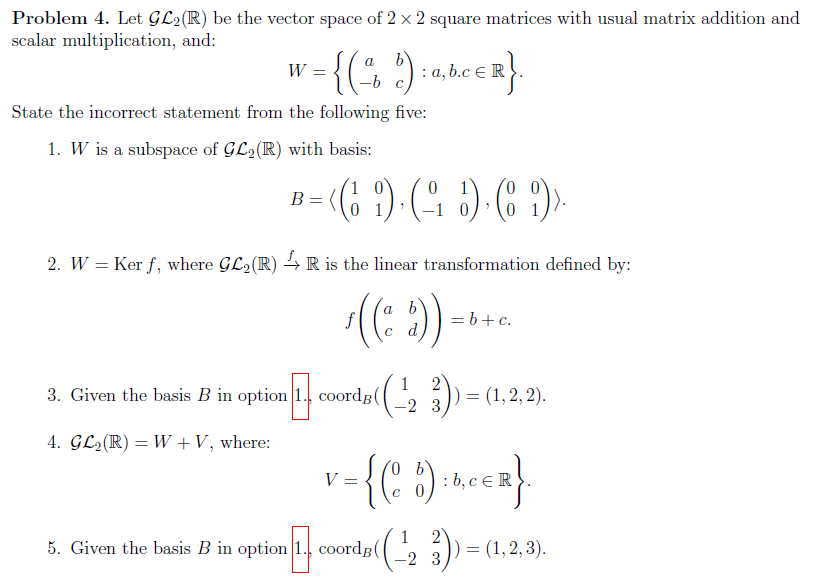Problem 4. Let GL2(R) be the vector space of 2 x 2 square matrices with usual matrix addition and scalar multiplication, and Wー State the incorrect statement from the following five 1. W is a subspace of GL2(R) with basis 2. W -Ker f, where GL2(R) R is the linear transformation defined by: 3. Given the basis B in option1. coordB( 23(1,2,2) 4. GC2(R)-W + V, where: 5. Given the basis B in option1. coordB( 2 3 (1,2,3) Problem 5....

Free Homework App